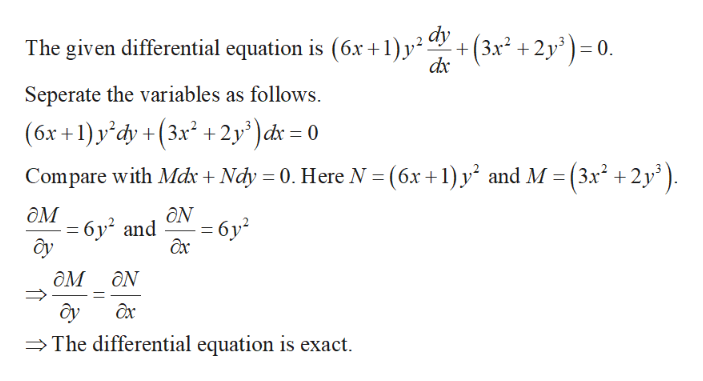# (6x+1)y^2y'+3x^2+2y^3=0

Question

(6x+1)y^2y'+3x^2+2y^3=0

check_circle

Step 1

Check whether the given differential equ...help_outlineImage Transcriptionclose+(3x2 2y30 dx The given differential equation is (6x +1)p2 dy Seperate the variables as follows (6x+1)y'dy +(3x2 +2y)d = 0 Compare with Mdx + Ndy 0. Here N (6x +1) y and M (3x2 +2y дм 6y2 and y - 6y2 ax ом Ох The differential equation is exact fullscreen

### Want to see the full answer?

See Solution

#### Want to see this answer and more?

Solutions are written by subject experts who are available 24/7. Questions are typically answered within 1 hour.*

See Solution
*Response times may vary by subject and question.
Tagged in

### Differential Equations